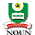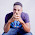# How To Calculate GPA and CGPA in National Open University of Nigeria

Today, I am going to share with you a short way to know your  GPA and CGPA at National Open University of Nigeria.. Many people get confused about the fact that they have perform poorly in the tenure at the school. so if you are the new comers. here is little things you need to know before you start gambling in the school campus.. Just sit Down and read it..
what is  GPA AND CGPA ?
GPA denotes Grade Point Average while CGPA denotes Cumulative Grade Point Average

The difference between the two lies in the fact that GPA is the standard of your academic performance in a particular semester while CGPA is the standard of your academic performance from the commencement of your programme till date.

CALCULATION OF GPA AND CGPA

I will use three courses to illustrate the technique in order to make it easier for you to understand.

After your TMAs and exams, your academic performance in all the courses you took in a particular semester is called GPA calculated thus:

Course 1:
Code. PHY 102
Points. 4 points
Units. 3 units
GP=3*4=12

Course 2:
Code. LAW 111
Points. 5 points
Units. 2 units
GP=5*2=10

Course 3:
Code. CIT 101
Points. 3 points
Units. 3 units
GP=3*3=9

GPA:

GP for PHY 102=12, GP for LAW 111=10 , GP for CIT 101=9

GPA=total GP/total credit units=12+10+9/3+2+3=31/8=3.88

If in the next semester, you obtain a GPA of 30/7, for instance, your CGPA at that time will be 31+30/8+7=61/15=4.07

CLASSES OF DEGREE

First Class (70-100) CGPA (4.5-5)

Second Class Upper (60-69) CGPA(3.5-4.49)

Second Class Lower (50-59) CGPA (2.4-3.49)

Third Class (45-49) CGPA(1.5-2.39)

##### 14 on: "How To Calculate GPA and CGPA in National Open University of Nigeria"
1.1.It can only be possible with this tool

2.2.3.1.Great Article. Thank you for sharing! Really an awesome post for every one.

IEEE Final Year projects Project Centers in Chennai are consistently sought after. Final Year Students Projects take a shot at them to improve their aptitudes, while specialists like the enjoyment in interfering with innovation. For experts, it's an alternate ball game through and through. Smaller than expected IEEE Final Year project centers ground for all fragments of CSE & IT engineers hoping to assemble. Final Year Project Domains for IT It gives you tips and rules that is progressively critical to consider while choosing any final year project point.

JavaScript Training in Chennai

JavaScript Training in Chennai

4.First of all, are they all regular courses (not AP/IB/Honors)? If so, then you calculate your GPA or CGPA through this Online CGPA Calculator:

-An "A" is worth 4 pts; "B" is 3 pts.; "C" is 2 pts.; etc.
-You have 5 A's and 3 B's
-Assuming you have all regular classes, let's add now
-so all together it's 29.
-then divide this number by the number of classes you have (which is 8)
-29/8= 3.625

HOWEVER, if you do have AP/IB/Honors classes, then the scale goes like this:
-A=5 pts. (instead of 4 pts like in a regular class)
-B=4 pts.
-and so on.

then, using these numbers, you can calculate your GPA by adding and dividing like I told you earlier.

5.Is the CGPA calculated for every two semesters or after your final year exam in the dschoo

6.When you finish a level e.g 100L are you going to be graded on first class,second class basic before you enter 200L

7.GPA Calculator app for students who wanted to know how College and High School GPA Calculator calculates your GPA. This easy to use GPA Calculator will keep you on track because you will always be aware of your current GPA. For more information, Please install GPA Calculator App.

8.9.10.11.